# DE102015215510A1 - Method for determining an orthogonality error between two sensor signals - Google Patents

Method for determining an orthogonality error between two sensor signals

## Info

Publication number
DE102015215510A1
DE102015215510A1 DE102015215510.2A DE102015215510A DE102015215510A1 DE 102015215510 A1 DE102015215510 A1 DE 102015215510A1 DE 102015215510 A DE102015215510 A DE 102015215510A DE 102015215510 A1 DE102015215510 A1 DE 102015215510A1
Authority
DE
Germany
Prior art keywords
correction value
angle
corrected
determining
rotation angle
Prior art date
Legal status (The legal status is an assumption and is not a legal conclusion. Google has not performed a legal analysis and makes no representation as to the accuracy of the status listed.)
Withdrawn
Application number
DE102015215510.2A
Other languages
German (de)
Inventor
Jens Gleißberg
Markus Irth
Current Assignee (The listed assignees may be inaccurate. Google has not performed a legal analysis and makes no representation or warranty as to the accuracy of the list.)
Continental Automotive GmbH
Original Assignee
Continental Automotive GmbH
Priority date (The priority date is an assumption and is not a legal conclusion. Google has not performed a legal analysis and makes no representation as to the accuracy of the date listed.)
Filing date
Publication date
Priority to DE102014216224.6 priority Critical
Priority to DE102014216224 priority
Priority to DE102014220331.7 priority
Priority to DE102014220331 priority
Application filed by Continental Automotive GmbH filed Critical Continental Automotive GmbH
Priority to DE102015215510.2A priority patent/DE102015215510A1/en
Publication of DE102015215510A1 publication Critical patent/DE102015215510A1/en
Withdrawn legal-status Critical Current

• 230000000737 periodic Effects 0.000 claims abstract description 7
• 230000001131 transforming Effects 0.000 claims description 11
• 230000004044 response Effects 0.000 claims description 2
• 238000004364 calculation methods Methods 0.000 description 14
• 238000010586 diagrams Methods 0.000 description 5
• 238000010606 normalization Methods 0.000 description 5
• 238000004088 simulation Methods 0.000 description 5
• 230000000694 effects Effects 0.000 description 4
• 238000004458 analytical methods Methods 0.000 description 2
• 238000006243 chemical reactions Methods 0.000 description 2
• 238000004519 manufacturing process Methods 0.000 description 2
• 239000007787 solids Substances 0.000 description 2
• 281000157896 Metaswitch companies 0.000 description 1
• 230000005540 biological transmission Effects 0.000 description 1
• 150000001875 compounds Chemical class 0.000 description 1
• 230000001419 dependent Effects 0.000 description 1
• 238000009795 derivation Methods 0.000 description 1
• 238000006073 displacement reactions Methods 0.000 description 1
• 238000009499 grossing Methods 0.000 description 1
• 238000000034 methods Methods 0.000 description 1
• 238000006467 substitution reactions Methods 0.000 description 1
• 230000001629 suppression Effects 0.000 description 1
• 230000001052 transient Effects 0.000 description 1

## Images

•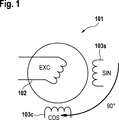•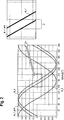•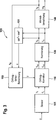•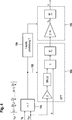•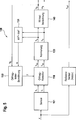•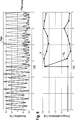••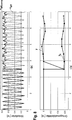## Classifications

• GPHYSICS
• G01MEASURING; TESTING
• G01DMEASURING NOT SPECIALLY ADAPTED FOR A SPECIFIC VARIABLE; ARRANGEMENTS FOR MEASURING TWO OR MORE VARIABLES NOT COVERED IN A SINGLE OTHER SUBCLASS; TARIFF METERING APPARATUS; MEASURING OR TESTING NOT OTHERWISE PROVIDED FOR
• G01D5/00Mechanical means for transferring the output of a sensing member; Means for converting the output of a sensing member to another variable where the form or nature of the sensing member does not constrain the means for converting; Transducers not specially adapted for a specific variable
• G01D5/12Mechanical means for transferring the output of a sensing member; Means for converting the output of a sensing member to another variable where the form or nature of the sensing member does not constrain the means for converting; Transducers not specially adapted for a specific variable using electric or magnetic means
• G01D5/244Mechanical means for transferring the output of a sensing member; Means for converting the output of a sensing member to another variable where the form or nature of the sensing member does not constrain the means for converting; Transducers not specially adapted for a specific variable using electric or magnetic means influencing characteristics of pulses or pulse trains; generating pulses or pulse trains
• G01D5/24471Error correction
• G01D5/24476Signal processing

## Abstract

The invention relates to a method for determining a corrected rotation angle (x_tl) of a raw rotation angle (x) detected by an angle sensor which outputs first and second raw rotation angle signals (s_r, c_r) as a function of the raw rotation angle (x) and which have a periodic progression orthogonal relationship to one another, wherein due to the error (y) may occur a deviation from the orthogonal relationship between the sensor signals, comprising the steps: - Determining a correction value (y) by means of a determination unit for determining the correction value (y) and providing the correction value (y ) to a correction unit, applying the correction value to at least one of the raw rotation angle signals (s_r, c_r) by means of the correction unit for determining at least one corrected rotation angle signal (s_oc, c_oc), and calculating the corrected rotation angle (x_tl) on the basis of at least one the corrected rotation angle signals (s_oc, c_oc).

## Description

• The invention relates to a method for determining a corrected angle of rotation of a raw angle of rotation detected by means of an angle sensor, an angle sensor arrangement and a drive device for carrying out such a method.
• From the prior art, the publication DE 10 2010 003 201 A1 in which a method for determining a rotation angle with an angle measuring unit is disclosed. In this document, it is disclosed how the rotation angle can be corrected by means of a correction value so that the influence of a false angle F on the value of the rotation angle is reduced. This is an error resulting from a not quite exact orthogonality between a sinusoidal and cosinusoidal sensor signal of the sensor element.
• In the priority application DE 10 2014 216 224.6 Further, there is disclosed a method which exhibits a possibility other than the above-mentioned document for determining the orthogonality error or a correction value for correcting the orthogonality error between the two angle signals.
• Proceeding from this, it is an object of the invention to show a possibility with which a corrected rotation angle calculation or determination can be carried out precisely and in a simple manner.
• The object is achieved according to a method of claim 1. Further advantageous embodiments of the method according to the invention are the subject of the dependent claims, which are expressly made by reference to the subject of the description.
• The invention was based on the basic concept of providing a method by means of which the correction value determination as well as the introduction of the correction value into the rotation angle calculation can be integrated as simply as possible into existing processes. On the basis of the method according to the invention, in particular the determination unit, a particularly flexible implementation of the error correction in an already set operation is possible. Furthermore, it is possible to adapt the possibilities for calculating or determining the correction value as required, for example, depending on the requirements for the accuracy of the calculation of the correction value. In contrast to the prior art, a determination unit for determining the correction value is used in addition to a correction unit. In this way, the manner in which the correction value is determined can be set flexibly in the determination unit.
• The method according to the invention is advantageously further developed in that corrected rotational angle signals for both raw rotational angle signals are corrected by means of the correction value and the corrected rotational angle is calculated by means of both corrected rotational angle signals. In this way it is ensured that both raw rotation angle signals are corrected in such a way that they essentially assume their desired value.
• The method according to the invention is advantageously further developed in that the corrected rotational angle signals are normalized to standardized rotational angle signals, preferably to a value range between -1 and 1. By means of normalization, the corrected rotational angle signals are processed so that the determination of the corrected rotational angle is independent of the scaling the Rohdrehwinkelsignale can be done. The inventive method can thus be applied to a variety of sensor types readily.
• According to the invention, a method for determining a corrected rotational angle (x_tl) of a raw rotational angle (x) or angular sensor signal detected by an angle sensor is proposed, which outputs first and second raw rotational angle signals (s_r, c_r) or sensor signals as a function of the raw rotational angle (x) have a periodic course and are in an orthogonal relationship to one another, whereby due to the error (y) a deviation from the orthogonal relationship between the sensor signals may occur, comprising the steps:
• Determining a correction value (y) by means of a determination unit for determining the correction value (y) and providing the correction value (y) to a correction unit,
• Applying the correction value to at least one of the raw rotation angle signals (s_r, c_r) by means of the correction unit for determining at least one corrected rotation angle signal (s_oc, c_oc), and
• - Calculating the corrected rotation angle (x_tl) based on at least one of the corrected rotation angle signals (s_oc, c_oc).
• The method according to the invention is advantageously further developed in that the corrected, preferably normalized, raw rotational-angle signals are continuously called up to determine the correction value. The so-called online determination of the correction value allows an even more precise determination of the correction value. The external effects on the Rohdrehwinkelsignale as well as other influences that lead to a change in Orthogonalitätsfehlers can be taken into account in this way continuously. Also, in this way, a vibration behavior of the Orthogonality error with in the correction value are taken into account. In particular, in applications in the automotive sector, for example in a steering assistance, this embodiment may be particularly advantageous to determine an accurate steering assist torque.
• The method according to the invention is further developed in a particularly advantageous manner in that the determination of the correction value comprises the steps:
• Forming a radius signal by means of the sums of squares of the corrected or normalized raw rotational-angle signals,
• - Determining the 2nd nth harmonic of the radius signal, with n equal to a whole positive number, and
• - Determining the error on the basis of a phase-shifted by 90 ° to the rotation angle value of the amplitude at the second harmonic.
• This way of determining the error correction is particularly easy to implement and has proven to be a particularly stable and accurate method for determining the error correction. It can be done both online and offline. The advantage of this embodiment lies in the fact that the error can be determined on the basis of the radius signal, which can be determined solely on the basis of the two raw rotational angle signals. Since these signals are necessary anyway for determining the angle of rotation, there is no need to change existing rotational angle sensors. There is no need for a reference sensor signal with which the sensor signals could be individually compared to determine the error in the individual sensor signals. The method can therefore be integrated particularly easily into existing systems, since the electronic means necessary for the evaluation of the sensor signals are present anyway.
• The mathematical derivation follows as follows. The amplitude of the radius signal is by means of the equation e_orth (x) = sin 2 (x) + cos 2 (x + y) can be mapped, where x is the value of the angle of rotation and y is the value of the error. If the error is y = 0, the aforementioned condition of the addition theorem is satisfied.
• Among other things, the amplitude of the radius signal has a maximum at an angle of 45 °, so that the radius signal assumes the following value at x = 45 °: e_orth (45 °) = 1 - sin (y)
• For the determination of e_orth (45 °) from the measured signals, other errors in the stereo output signal e_orth (x) or e must be hidden. This can be done by harmonic analysis using Fourier transform. In this case, the magnitude of the imaginary part of the 2nd harmonic as a result of the Fourier transformation corresponds to the value e_orth (45 °). The advantage here is the complete suppression of other errors in the signal e_orth (x) by the sole consideration of the second harmonic, such as offset, gain or Deachsierungsfehlern because they do not affect the 2nd harmonic. Since, as a result of the Fourier transformation, the amplitudes are calculated, but y describes the peak-to-peak value (from the minimum value to the maximum value), the magnitude of the second harmonic imaginary part must be doubled: y = arcsin [2 · (e_orth, 2 · n., im)]
• The method according to the invention is advantageously further developed in that the second or 2 × n-th harmonic of the radius signal is determined by means of a Fourier transformation, preferably discrete Fourier transformation. The discrete Fourier transformation makes it possible to perform the aforementioned method with as little computation as possible.
• The method according to the invention is advantageously further developed by using the imaginary component of the second or 2nth harmonic of the radius signal to calculate the correction value. This embodiment is based on the idea that the error has an immediate effect on the amplitude of the second or an integral multiple of the second harmonic of the radius signal and therefore an analysis of the amplitude of the second harmonic gives a direct indication of the magnitude of the error. In particular, this embodiment is based on the recognition that the error occurs in the second harmonic of the radius signal with a phase shift of 90 ° to the rotation angle, so that the imaginary part of the harmonic results in an exclusion over the error.
• The method according to the invention is advantageously further developed in that the correction value is calculated using the equation y = Σ [arcsin (2 · e_2 · n, im)] or preferably based on y = Σ [2 · e_2 · n, im] where e_2 * n, im is the value of the imaginary portion of the second or 2nth harmonic. Especially with the latter simplification, a possibility is shown with which the computational effort for determining the correction value can be further reduced. It is based on the assumption that The correction value is usually within a small range of values around zero and thus leads this assumption for a variety of applications to a simplified calculation.
• The method according to the invention is advantageously further developed in that the value of the imaginary part of the harmonic wave is determined only at intended rotational angles (x_ST). In this way it is ensured that within one revolution the imaginary part of the 2nd or 2nd n th harmonic is not calculated several times for an angle.
• The method according to the invention is advantageously further developed in that the values of the imaginary part of the harmonic at the angles of rotation x_ST = {0, 1, 1 × 2π / N, 2 × 2π / N, ..., (N-1) × 2π / N} is calculated, where N is a positive integer. This fixes the calculation of the imaginary component to fixed angles or angular positions. This has the advantage that the calculation of the imaginary component can be determined independently of the speed at which the angle of rotation changes.
• The method according to the invention is advantageously further developed in that the correction value is set once for each sensor. The so-called offline calculation or determination of the correction value is low in effort due to the one-time calculation and can also be carried out by means of external computing units, whereby production costs of sensor arrangements can be kept low.
• The object is also achieved by means of an angle sensor arrangement having a sensor unit for detecting the raw rotational-angle signals and an evaluation unit for carrying out a method according to one of the aforementioned embodiments.
• Furthermore, the object is also achieved by means of a drive device with an electric motor, in particular for a steering assistance device, a control device for controlling the electric motor, and with an angle sensor arrangement according to the aforementioned embodiment.
• The invention will be described below with reference to an embodiment and with reference to several figures. Show it:
• 1 a basic structure of an angle sensor for an angle sensor arrangement,
• 2 an exemplary representation of an orthogonality error in the angle sensor,
• 3 a block diagram of an angle sensor arrangement for carrying out the method according to the invention,
• 4 a detailed representation of a determination unit from the block diagram from 3 .
• 5 a block diagram for performing a comparison test between an absolute target angle value and the corrected angle of rotation, and
• 6 - 8th a comparison of the results with and without rotation angle correction.
• Some reference numerals in the figures each have a small index, which will be described in the following description by way of substitution of the character "".
• 1 shows the structure of an angle sensor 101 , the Indian 3 shown angle sensor arrangement 100 is used. The angle sensor 101 has an angle encoder 102 , The angle encoder 102 specifies a rotation angle signal x, that of two sensor elements 103s . 103c be recorded. Each sensor element 103s . 103c generated from the angle of rotation signal x of the angle encoder 103 a raw rotation angle signal s_r and c_r, which is used for calculating or for determining the corrected rotation angle x_tl.
• The raw rotation angle signals s_r, c_r are a periodic signal, for example, a sine and a cosine signal, which are shifted by 90 ° to each other phases. Due to the orthogonal relationship between the sensor signals, the sensor signals should satisfy the condition according to the addition theorem sin 2 (x) + cos 2 (x) = 1, with x as the value of the rotation angle. Due to different causes, the example. Once in the production of the angle sensor 102 can occur or external influences in the operation, which are permanently present, there may be a deviation of the orthogonal relationship between the two raw rotation angle signals s_r and c_r. In 2 an error case is shown by way of example in which the actual raw rotational angle signal s_r has an orthogonality error and therefore erroneously assumes the course of the curve s_r_err. As a result, the phase shift between the two Rohdrehwinkelsignalen not 90 ° but 90 ° + y. The inventive method allows to determine this error as a correction value y.
• In 3 is an angle sensor arrangement 100 shown with the method of the invention can be performed. The angle sensor arrangement comprises, on the one hand, the angle sensor 101 , This is with a correction unit 110 connected. The correction unit 110 is in turn with one normalization unit 130 connected. The normalization unit is equipped with an angle calculation unit 140 connected. Furthermore, the angle sensor arrangement comprises 100 a determination unit 150 to determine the correction value y. The determination unit 150 is with the correction unit 120 connected and provides this the correction value y available. Connected in the sense of the embodiment means a connection for the transmission of signals or data. Preferably, this is a data connection. In the figures, the compounds are each represented by an arrow. It is not necessarily necessary for the angle sensor arrangement to be a normalization unit 140 having. This is advantageous, however.
• The method is carried out in such a way that, on the one hand, the determination unit 150 determines a correction value y and this value to the correction unit 120 provides. The correction value is applied to at least one of the raw rotation angle signals s_r, c_r by means of the correction unit for determining at least one corrected rotation angle signal s_oc, c_oc, from which the corrected rotation angle x_tl is calculated on the basis of at least one of the corrected rotation angle signals s_oc, c_oc. In this exemplary embodiment, corrected rotational angle signals s_oc, c_oc are determined for both raw rotational-angle signals s_r, c_r by means of the correction value (y), and the corrected rotational angle x_tl is calculated by means of both corrected rotational-angle signals s_oc, c_oc. Further, this embodiment also includes the step of normalizing the corrected rotation angle signals s_oc, c_oc to normalized rotation angle signals s_n, c_n. The normalization is preferably carried out in such a way that the standardized rotation angle signals s_c, c_n are then within a range of values between -1 and 1.
• Furthermore, the exemplary embodiment has the property that the corrected and normalized raw rotational angle signals s_n, c_n are continuously retrieved for determining the correction value. For this purpose, as in 3 see, the normalized rotation angle signals s_n, c_n to a computing unit 151 in which a radius signal e is calculated by means of the equation s_n 2 + c_n 2 . Based on the radius signal then the correction value y in the determination unit 150 calculated or determined. In 4 is the structure of the determination unit 150 shown closer.
• The determination unit 150 includes on the one hand DFT block 152 and on the other hand, an integration block 153 ,
• Within the DFT block 152 the second harmonic is calculated from the radius signal e by means of a discrete Fourier transformation. Since only the imaginary component is decisive for determining the orthogonality error or the correction value y, the calculation can be further simplified. Ie. instead of calculating over the equation can perform the calculation by the equation be further specified.
• However, this is done only for certain angular positions or angles. For this purpose, the current corrected rotation angle x_tl is retrieved and a check 154 performed, whether the current corrected angle of rotation x_tl corresponds to a stored in a memory predetermined rotation angle x_ST. In a first pass, the corrected rotation angle x_tl can also be uncorrected. Preferably, the values of the predetermined rotation angle lie at interpolation points x_ST, which may be defined as follows: x_ST = {0, 1, 1 × 2π / N, 2 × 2π / N, ..., (N-1) × 2π / N}
• If this is the case, the calculation of the summand e ^ _2, is performed in what is indicated by the arrow 155 is illustrated. The calculation of the sinusoidal signal can also be replaced by the use of a table with the sine values matching the position x_ST.
• From the imaginary part of the second harmonic e ^ _2, im can then use the equation to generate a single correction value y_s = arcsin (2 · e ^ _2, im) be calculated. On the assumption that the values of the imaginary part of the second harmonic are close to zero, a simplification can also be made, namely y_s = 2 · e ^ _2, im
• For the compensation of the orthogonality error, the value y must be the sum y = y + y_s be formed, since upon feedback of the specific Kompensationswert y an orthogonality error of y_s = 0 would result. The initial value of y is 0. To avoid sudden jumps in the angle signal x_tl, the signal y should have a change limitation, which can be easily realized, for example, by limiting the value of y_s. The effectiveness of the method according to the invention is described below in the 5 to 8th shown.
• 5 shows a structure with which a simulation of the inventive method was performed. The structure essentially corresponds to the structure of the angle sensor arrangement 100 , To compare the effectiveness of the angle sensor arrangement 100 or the method according to the invention, the value of a reference sensor x_ref was used in order to make a comparison between the value of the reference sensor x_ref and the corrected rotation angle x_tl.
• The raw angles of rotation x were made on real resolvers with subsequent simulation of the angle error, d. H. the angle error was added artificially. The signals x_ref, x_tl, s_r and c_r were recorded. Different orthogonality errors were used, as described below.
• During the simulation, the s_r and c_r signals are fed into the model as stimuli. Thus, two systems for angle calculation are realized, which receive exactly the same input data. On the one hand, the model simulation with automatic orthogonality compensation by means of the correction value y. In addition, a control unit without orthogonality compensation was used for comparison.
• The angle x_tl calculated by the model can be compared with the reference angle x_ref and forms the angle difference x_diff, comp. In addition, the angle error without orthogonality compensation is calculated from the measured values of the control unit as x_diff for comparison. Both systems will settle for about 3.2 seconds before the simulation performs automatic orthogonality correction. Thus, the effect can be visualized visually.
• The 6 . 7 and 8th show the results for resolvers with injected orthogonality errors
• - 6 : 0.154 ° el
• - 7 : 0.655 ° el
• - 8th : -0.3 ° el.
• The upper diagram shows the angle error without compensation x_diff as a red / dashed line. The compensated value x_diff_comp is shown as a blue / solid line. At the time t = 3.75 s, the first value for the orthogonality error was determined and fed to the orthogonality compensation. The effect is clear to see, the angle error has no dominant 2nd order more.
• In the lower diagram, the signal y as the determined orthogonality error is shown as a green / solid line, and the signal y_s as a blue / dashed line as the determined_ residual orthogonality error.
• In the 6 to 8th you can see the significant reduction of the orthogonality error. In the application shown here, the remaining angle error no longer has a critical size.
• The compensation value y was directly applied without smoothing, which makes it possible to use in particular 7 Angular jumps have occurred in the change of y. For this, one can easily see how y_s once assumes the value of y in order then to remain at the level of recognition accuracy. If you smooth y, the signals y and y_s show a transient response.
• Note that the compensation value is the peak-to-peak value of the harmonic angle error. It has twice the value of y_s = arcsin (e_2, im). Furthermore, it can be clearly seen that the correction of the orthogonality error eliminates the angular displacement in the amount of orthogonality error.
• Below, further advantageous variants are listed, which can be combined with the aforementioned embodiments:
• 1. A method for determining an error (y) between two sensor signals (s1, s2) in an angle sensor which outputs the sensor signals (s1, s2) in dependence on an angle sensor, which have a periodic course and are mathematically in an orthogonal relationship to each other, wherein, due to the error (y), a deviation from the orthogonal relationship between the sensor signals may occur, comprising the steps of:
• Forming a radius signal (e_orth) by means of the sums of squares of the sensor signals,
• - Determining the 2 nth harmonic of the radius signal (e_orth), with n equal to a whole positive number, and
• - Determining the error of a phase-shifted by 90 ° to the angle of rotation value of the amplitude at the second harmonic.
• 2. The method according to claim 1, characterized by
• Determining a frequency component or portions of the radius signal by means of a Fourier transformation, and
• - Determine the error based on the imaginary part of the frequency component or the frequency components of the second harmonic.
• 3. The method according to claim 2, characterized in that the Fourier transformation is performed by means of a fast and / or discrete Fourier transformation.
• 4. The method of claim 2 or 3, characterized by, calculating the error y by means of the equation y = arcsin | e_orth, 2 · n., in | is determined, where e_orth, 2nd, im in the imaginary part of the amplitude of the 2 · n-th harmonic of the radius signal maps.
• 5. The method according to any one of the preceding claims, characterized in that the real part of the 2 nth harmonic wave is used as a scale for a scaling error.
• 6. The method according to any one of the preceding claims, characterized in that the method is used during operation.
• 7. The method according to any one of claims 1-5, characterized in that the method is carried out before commissioning of an angle sensor, in particular by means of an external computing unit.
• 8. A method for determining an error (y) between two sensor signals (s1, s2) in an angle sensor which outputs the sensor signals (s1, s2) in dependence on an angle sensor, which have a periodic course and are mathematically in an orthogonal relationship to each other, wherein, due to the error (y), a deviation from the orthogonal relationship between the sensor signals may occur, comprising the steps of:
• Determining a rotation angle (x) from the sensor signals (s1, s2),
• Determining a conversion value by performing a Fourier transformation for the determined rotation angle,
• - Determine the error based on the conversion value.
• 9. An angle sensor for detecting a rotation angle, comprising
• A sensor element that outputs the sensor signals as a function of an angle sensor, which have a periodic course and are mathematically in an orthogonal relationship to one another, and
• - A computing unit for carrying out the method according to any one of the preceding claims.
• QUOTES INCLUDE IN THE DESCRIPTION
• This list of the documents listed by the applicant has been generated automatically and is included solely for the better information of the reader. The list is not part of the German patent or utility model application. The DPMA assumes no liability for any errors or omissions.
• Cited patent literature
• DE 102010003201 A1 
• DE 102014216224 

## Claims (14)

1. Method for determining a corrected angle of rotation (x_tl) of a raw angle (x) detected by means of an angle sensor which outputs first and second raw angle signals (s_r, c_r) which have a periodic progression and are in an orthogonal relationship as a function of the raw angle of rotation (x) in which, due to the error (y), a deviation from the orthogonal relationship between the sensor signals may occur, comprising the steps: Determining a correction value (y) by means of a determination unit for determining the correction value (y) and providing the correction value (y) to a correction unit, Applying the correction value to at least one of the raw rotation angle signals (s_r, c_r) by means of the correction unit for determining at least one corrected rotation angle signal (s_oc, c_oc), and - Calculating the corrected rotation angle (x_tl) based on at least one of the corrected rotation angle signals (s_oc, c_oc).
2. A method according to claim 1, characterized in that for both Rohdrehwinkelsignale (s_r, c_r) by means of the correction value (y) corrected rotational angle signals (s_oc, c_oc) are determined and the corrected rotational angle (x_tl) by means of both corrected rotational angle signals (s_oc, c_oc) is calculated ,
3. A method according to claim 1 or 2, characterized by normalizing the corrected rotational angle signals (s_oc, c_oc) to normalized rotational angle signals (s_n, c_n), preferably to a range of values between -1 and 1.
4. Method according to one of the preceding claims, characterized in that the corrected, preferably normalized, raw rotational angle signals (s_oc, c_oc / s_n, c_n) are continuously retrieved for determining the correction value.
5. A method according to claim 3, characterized in that the corrected rotation angle signals (s_oc, c_oc) or the normalized rotation angle signals (s_n, c_n) in response to the corrected rotation angle (x_tl) for determining the correction value (y) are retrieved.
6. Method according to one of the preceding claims, characterized in that the determination of the correction value comprises the steps of: - forming a radius signal (e) by means of the sums of squares of the corrected or normalized raw rotation angle signals (s_oc, c_oc / s_n, c_n), - determining the 2 · n -th harmonic of the radius signal (e), with n equal to a whole positive number, and - determining the error by means of a phase-shifted by 90 ° to the rotation angle (x) value of the amplitude at the second harmonic.
7. A method according to claim 5, characterized in that the second or 2nd nth harmonic of the radius signal (e) is determined by means of a Fourier transformation.
8. Method according to Claim 6, characterized in that the imaginary component of the second or 2 · nth harmonic of the radius signal (e) is used to calculate the correction value.
9. Method according to one of claims 5 to 8, characterized in that the correction value based on the equation y = Σ2 · arcsin (e_2 · n, im) or preferably based on y = Σ2 · e_2 · n, im where e_2 * n, im is the value of the imaginary portion of the second or 2nth harmonic.
10. Method according to one of claims 6 or 7, characterized in that the value of the imaginary portion of the harmonic wave is determined only at intended rotation angles (x_ST).
11. A method according to claim 9, characterized in that the values of the imaginary part of the harmonic at the angles of rotation x_ST = {0, 1, 1 × 2π / N, 2 × 2π / N, ..., (N-1) × 2π / N} is calculated, where N is a positive integer.
12. Method according to one of claims 1 to 3 and 5 to 8, characterized in that the correction value (y) is set once for each sensor.
13. Angle sensor arrangement with a sensor unit for detecting the Rohdrehwinkelsignale (s_r, c_r) and an evaluation unit for carrying out a method according to one of claims 1 to 11.
14. Drive device with an electric motor, in particular for a steering assistance device, a control device for controlling the electric motor, and with an angle sensor arrangement according to claim 12.
DE102015215510.2A 2014-08-14 2015-08-13 Method for determining an orthogonality error between two sensor signals Withdrawn DE102015215510A1 (en)

## Priority Applications (5)

Application Number Priority Date Filing Date Title
DE102014216224.6 2014-08-14
DE102014216224 2014-08-14
DE102014220331.7 2014-10-07
DE102014220331 2014-10-07
DE102015215510.2A DE102015215510A1 (en) 2014-08-14 2015-08-13 Method for determining an orthogonality error between two sensor signals

## Applications Claiming Priority (1)

Application Number Priority Date Filing Date Title
DE102015215510.2A DE102015215510A1 (en) 2014-08-14 2015-08-13 Method for determining an orthogonality error between two sensor signals

## Publications (1)

Publication Number Publication Date
DE102015215510A1 true DE102015215510A1 (en) 2016-02-18

# Family

## Family Applications (1)

Application Number Title Priority Date Filing Date
DE102015215510.2A Withdrawn DE102015215510A1 (en) 2014-08-14 2015-08-13 Method for determining an orthogonality error between two sensor signals

## Country Status (6)

US (1) US20170153127A1 (en)
EP (1) EP3180592A1 (en)
KR (1) KR20170029608A (en)
CN (1) CN106574851A (en)
DE (1) DE102015215510A1 (en)
WO (1) WO2016024001A1 (en)

## Citations (3)

* Cited by examiner, † Cited by third party
Publication number Priority date Publication date Assignee Title
DE10163504A1 (en) * 2001-12-21 2003-07-10 Siemens Ag Method for iterative error compensation of a sine-cosine position measurement system for application to offset, amplitude and phase errors, so that almost exact correction values can be determined
DE102004038621B3 (en) * 2004-08-09 2006-02-16 Siemens Ag Determination procedure for a position signal
DE102010003201A1 (en) 2009-05-08 2010-12-23 Continental Teves Ag & Co. Ohg Angle-measuring method for determining an angle of tilt/swiveling angle uses an angle-measuring unit and first and second measuring signals

## Family Cites Families (8)

* Cited by examiner, † Cited by third party
Publication number Priority date Publication date Assignee Title
US5463393A (en) * 1991-12-05 1995-10-31 Acutronic Ag Method and apparatus for correcting errors in an amplitude encoded signal
WO2005050140A1 (en) * 2003-11-18 2005-06-02 Koninklijke Philips Electronics N.V. Position determining
JP4713123B2 (en) * 2004-10-13 2011-06-29 株式会社ミツトヨ Encoder output signal correction device
JPWO2006043403A1 (en) * 2004-10-20 2008-05-22 株式会社安川電機 Encoder signal processing apparatus and signal processing method thereof
JP4568298B2 (en) * 2007-03-16 2010-10-27 オークマ株式会社 Position detection device
JP5178374B2 (en) * 2008-07-29 2013-04-10 キヤノン株式会社 Detection device
JP2010156554A (en) * 2008-12-26 2010-07-15 Okuma Corp Position detecting apparatus
JP5836026B2 (en) * 2011-09-08 2015-12-24 三菱重工業株式会社 Error frequency component acquisition device, rotation angle acquisition device, and motor control device

## Patent Citations (3)

* Cited by examiner, † Cited by third party
Publication number Priority date Publication date Assignee Title
DE10163504A1 (en) * 2001-12-21 2003-07-10 Siemens Ag Method for iterative error compensation of a sine-cosine position measurement system for application to offset, amplitude and phase errors, so that almost exact correction values can be determined
DE102004038621B3 (en) * 2004-08-09 2006-02-16 Siemens Ag Determination procedure for a position signal
DE102010003201A1 (en) 2009-05-08 2010-12-23 Continental Teves Ag & Co. Ohg Angle-measuring method for determining an angle of tilt/swiveling angle uses an angle-measuring unit and first and second measuring signals

## Also Published As

Publication number Publication date
KR20170029608A (en) 2017-03-15
WO2016024001A1 (en) 2016-02-18
US20170153127A1 (en) 2017-06-01
EP3180592A1 (en) 2017-06-21
CN106574851A (en) 2017-04-19

## Similar Documents

Publication Publication Date Title
DE102015121717B4 (en) Electric power steering system for a vehicle
CN102986132B (en) Current sensor error compensation
WO2017104674A1 (en) Motor module and motor authentication method
EP1315954B1 (en) Method for determining an angle differential from phase signals
JP2515891B2 (en) Angle sensor, torque sensor, and electric power steering device controlled according to the outputs of the sensors
CN101652636B (en) Magnetic encoder and method of detecting absolute rotational position
US8723511B2 (en) Absolute encoder
CN102594230B (en) Rotary angle detecting device
JP5725377B2 (en) Rotation angle detector
EP1508783B1 (en) Rotational angle detector and its temperature correcting method
EP1647811B1 (en) Encoder output signal correction apparatus and method
JP2007198942A (en) Rotary encoder
JP2012145488A (en) Abnormality detecting device for resolvers
JP2007518110A (en) Integrated non-contact torque and absolute position sensor for steering applications
JP6163874B2 (en) Rotation angle detection device, image processing device, and rotation angle detection method
EP2466268B1 (en) Rotation angle detection device
JP5097858B2 (en) Resolver digital converter
KR20120059956A (en) An apparatus for adaptively compensating position error of resolver
EP1804032A1 (en) Encoder signal processor and processing method
JP5720932B2 (en) Rotation angle detector
JP2010164426A (en) Rd converter and angle detecting apparatus
JP4979352B2 (en) Resolver / digital converter and control system using the resolver / digital converter
US20070090831A1 (en) Rotation angle detecting device having function of detecting abnormality
Funk et al. Indirect determination of the displacement components in an electric motor drive
KR100882400B1 (en) Phase correction circuit of encoder signal

## Legal Events

Date Code Title Description
R163 Identified publications notified
R119 Application deemed withdrawn, or ip right lapsed, due to non-payment of renewal fee